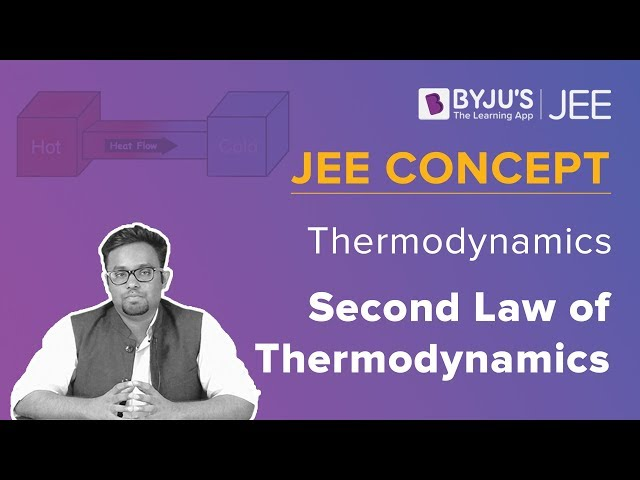# Heat Engine

Heat is a form of energy that can be easily transferred from one body to another. Heat Engine is one of the most interesting concepts in Physics. In this article, let us discuss different parts of the heat engine and its various types.

## What is Heat Engine?

A heat engine is a device that converts the energy locked in fuel into force and motion. Fuels like coal, gasoline, natural gas, wood, and peat when burnt in an engine, release the energy it contains to power factory machinery and locomotives. As engines work by burning fuels to release heat, they are called heat engines. Hence, heat engine can be defined as,

An engine that converts the chemical energy of the fuel into thermal energy which is utilised to do useful work

Heat engines are categorized into two types as follows: external combustion engine and internal combustion engine. In the next section, let us look at the two types in detail.

## Types of Heat Engine

As discussed, heat engines are classified into two main types as,

• ### Internal Combustion Engines

This process includes the combustion of a fuel that takes place within the system. These types of engines take place where the fuel is burnt in the engine or where the fossil fuel combustion occurs. Pistons are mostly used in the internal combustion type of heat engines. These pistons move up and down within the cylinders that are present in the heat engines. When a single motion of a piston move in the upward or downward direction inside the cylinder is known as the stroke. For Example – Mostly Cars have four-stroke internal combustion heat engines that consist of an Intake stroke, power stroke, combustion stroke, and exhaust stroke.

• ### External Combustion Engines

These type of heat engines takes where the fuel is burnt outside the engine or where the fuel combustion occurs outside the engine. It is a heat engine where a working fluid is included internally and heated by combustion in an external source through the engine wall. This fluid then produced motion and usable work by expanding and acting on the mechanism of the engine.

## Parts of Heat Energy

Heat energy is composed of three parts:

• Working object
• Source of heat at high temperature
• Sink of heat at a lower temperature• Low initial cost.
• Simple and easy design.
• Easily used in cold conditions.
• Produces comparatively higher power output per unit weight of the fuel.
• Energy loss is minimal.

• Cannot be operated with solid fuels like coal.
• High maintenance.

• Compatible with most type of fuels
• Economical
• Can be operated in high altitude places
• Emissions are low.

• Requires more space
• High maintenance.
• Operation temperature is very high.

Know about the second law of thermodynamics and operation of the heat engine from the below video.### How does a heat engine power a machine?

A basic heat engine consists of a gas confined by the piston in a cylinder. When the gas is heated, it expands and moves the piston. This wouldn’t be possible in a practical engine because the motion stops once the gas reaches equilibrium. A practical engine goes through cycles in which the piston moves back and forth. When the gas gets heated, the piston moves upwards and when it is cooled it moves downward. A cycle of heating and cooling is necessary to make the piston move forward and backward.

In a full cycle of heat engine, three things happen as follows:

• Heat is added at a relatively high temperature, hence it can be called QH
• Some part of the added energy is used to perform work
• The unused energy is removed at a relatively cold temperature QC

The diagram below is a representation of heat showing the energy flow.

An important measure of a heat engine is its efficiency. The efficiency of a heat engine depends on the ratio of the work obtained to the heat energy in the high temperature i.e. e = W/Q high. The maximum possible efficiency e max of an engine is

emax =Wmax/Qhigh = (1 – Tlow / Thigh) = (Thigh – Tlow)/Thigh

### How to find the efficiency of a heat engine?

Example –  If a gasoline engine has an efficiency of 30%, what would be the hot reservoir temperature for a Carnot engine having that Engine efficiency if it runs with a cold reservoir temperature of 200 ºC?

Solution  – Maximum efficiency of a Carnot engine e max = (Thigh – Tlow)/Thigh

If emax = 0.3, then 0.3 = 1 – (473 K)/Thigh

Thigh = 473/0.7 = 675.7 K = 402.7 oC

## Frequently Asked Questions – FAQs

Q1

### What is a heat engine?

Engine that converts the chemical energy of the fuel into thermal energy which is utilised to do useful work is known as a heat engine.

Q2

### What are the types of heat engines?

The following are the types of heat engine:

• Internal Combustion Engines
• External Combustion Engines
Q3

### What are the parts of a heat engine?

Following are the parts of a heat engine:

• Working object
• Source of heat at high temperature
• Sink of heat at a lower temperature
• Q4

### The maximum possible efficiency e max of an engine is given by which formula?

The maximum possible efficiency e max of an engine is given by the formula – emax =Wmax/Qhigh = (1 – Tlow / Thigh) = (Thigh – Tlow)/Thigh
Q5

### State true or false: Measure of a heat engine is its efficiency.

TRUE

Hope you have understood the types of heat engine along with parts of the heat engine. Stay tuned with BYJU’S to learn various science and math concepts in an interesting way.

Test your knowledge on Heat engine